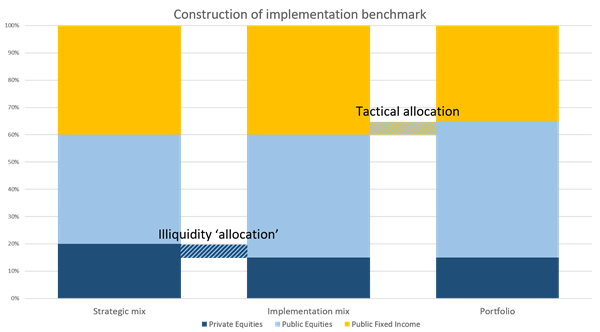In the current low-yield environment institutional investors allocate increasingly to illiquid assets. However, illiquid assets pose additional challenges when it comes to performance measurement and attribution. This insight explains how a portfolio can be evaluated fairly after the inclusion of illiquid assets in the strategic asset mix.

### Case description

Assume that the board sets target asset allocations of 40%, 40% and 20% to public equity, (public) fixed income, and private equity, respectively. The 20% target weight for private equity however, is not replicable as allocation in or out of the illiquid asset category is not at the discretion of the asset owner. Assume that, in addition, the investment committee decides upon a tactical bet to overweight equity (+5%) and underweight fixed income (-5%). The resulting portfolio weights (and therefore the calculated ex-post allocation results) are now a mixture of the tactical bet and the inability to allocate at target to the illiquid asset category.

### Implementation benchmark

In order to circumvent this issue, one should define an implementation benchmark which assumes the target weights that have been set by the board, but account for the illiquid allocation issue. We have seen and implemented many different ways to do this. The particular strategy of choice depends on the strategic investment beliefs of the institutional investor. All strategies have in common that they set the benchmark weight for the illiquid asset category equal to the portfolio weights. In addition, the capital invested relative to the board's target weights is drawn from one or more liquid asset categories.

### Example

An implementation benchmark example would assume a total equity weight of 60% (sum of public and private as set by the board). The private equity weight would equal the portfolio weight and public equity would make up the remainder. Assume that the portfolio has a 15% weight in private equity, and the allocation-neutral weight to public equity becomes 45%.### Analysis

The implementation benchmark can be compared with the portfolio to properly evaluate the tactical allocation bet among the liquid asset categories. Finally, the difference between the implementation benchmark and the strategic asset mix can be attributed to the inability to allocate at target in the illiquid asset categories.

### Interpretation

Unlike other attribution effects that show in a macro/decision attribution context, this effect - due to the inability to invest - is not a conscious decision in the investment process and therefore not attributable to any decision. It merely represents the realized result on a risk factor that has been introduced with the illiquid nature of parts of the strategic asset mix.

### Conclusion

Needless to say, there is much more to take into account when illiquid asset categories are part of the strategic asset mix. The framework described above will help to separate excess result of actual investment decisions from result that arises because of the nature of illiquid investments. In case you’d like to learn more about the topic, please contact .